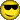# Translating a task into an assembly instructionClassicListThreaded4 messagesOpen this post in threaded view
|

## Translating a task into an assembly instruction

 This post was updated on . Hi all. Or probably WBahnI'm trying to translate the following task as you might do in a higher-level language into assembly instruction: arr[j] = 17; I was able to find a solution to a similar problem where I have to translate arr[j] = 0 or arr[j] = -1 I cannot assume to know anything about arr except that its ROM instruction references some memory location in the RAM, which is the 0 index of the array. j assumes M[j] has a value stored, i.e. M[j] = some integer My translation for arr[j] = 0 is as follows: @arr            // arr is a reference to some ROM location containing the instruction @n, so that A = n D = A          // D = n, where n is the memory location representing index 0 of the 'array' arr@j              // j is a reference to some ROM location containing the instruction @c, so that A = c D = D + M    // D = n + M[c], so that D is the memory location representing index j of the 'array'arrA = D         // We want to change the value stored at RAM[D], but Hack only allows calls to RAM[A],                 // so A must equal D so that, at least symbolically, RAM[A] == RAM[D] M = 0        //As per the c instruction, dest = M[A], and comp = 0 The problem is that this only works because the C instruction allows direct assignment of M to either 0, 1, or -1. If I want to translate arr[j] = 17, I can't simply call M = 17. I also can't store 17 in D, because the following happens: @17 D = A // I now need to get the instructions located at ROM[arr] and ROM[j] @arr // A now equals the memory location where the array begins, so now I only need to add j @j // I've now lost the memory location where the array begins and traded it for the memory location containing the index j. Which is a problem. I've been thinking about this problem for a long time but I can't figure it out!!!! Any help is greatly appreciated.
Open this post in threaded view
|

## Re: Translating a task into an assembly instruction

 Administrator This post was updated on . Let's tackle the simple one and see if that gives you ideas for how to proceed with the bigger problem. High level task: x = 42; In Hack assembly, we have to always be aware that we have extremely limited resources. We have the A and the D register and the A register has to do double-duty as both a second data register and as an address register. So we have to sequence things very carefully. So how about this: 1) Get the value we want to store into the D register and then be careful not to do anything to mess this up. 2) Get the address where we want to store the value into the A register. 3) Store the contents of D into the RAM location referenced by A.